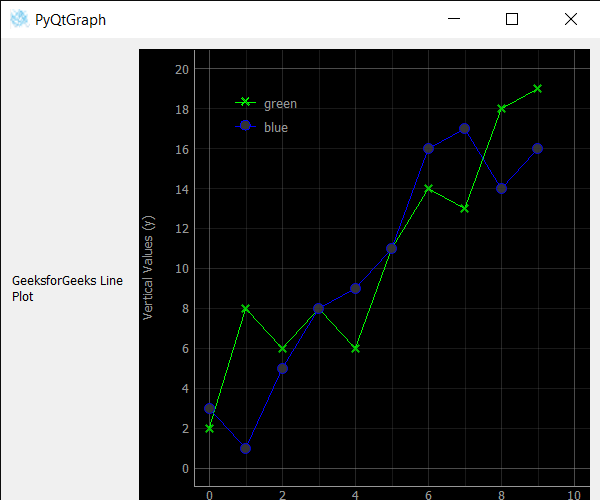# PyQtGraph – Setting Symbol Brush of Line in Line Graph

In this article we will see how we can set symbols brush of line in line graph of the PyQtGraph module. PyQtGraph is a graphics and user interface library for Python that provides functionality commonly required in designing and science applications. Its primary goals are to provide fast, interactive graphics for displaying data (plots, video, etc.) A line chart or line plot or line graph or curve chart is a type of chart which displays information as a series of data points called ‘markers’ connected by straight line segments. It is a basic type of chart common in many fields. Line graph is created with the help of plot class in PyQtGraph. Symbols are the figure of the point which connect the data of the line. It is mainly x, o.

We can create a plot window and create lines on it with the help of commands given below

```# creating a pyqtgraph plot window
plt = pg.plot()

# ploting line in green color
# with dot symbol as x, not a mandatory field
line = plt.plot(x, y, pen='g', symbol='x', symbolPen='g', symbolBrush=0.2, name='green')
```

In order to do this we use `setSymbolBrush` method with the line object

Syntax : line.setSymbolBrush(QColor(10, 130, 3))

Argument : It takes Color/brush object as argument

Return : It returns None

Below is the implementation

 `# importing Qt widgets ` `from` `PyQt5.QtWidgets ``import` `*` `import` `sys ` ` `  `# importing pyqtgraph as pg ` `import` `pyqtgraph as pg ` `from` `PyQt5.QtGui ``import` `*` ` `  `# Bar Graph class ` `class` `BarGraphItem(pg.BarGraphItem): ` ` `  `    ``# constructor which inherit original ` `    ``# BarGraphItem ` `    ``def` `__init__(``self``, ``*``args, ``*``*``kwargs): ` `        ``pg.BarGraphItem.__init__(``self``, ``*``args, ``*``*``kwargs) ` ` `  `    ``# creating a mouse double click event ` `    ``def` `mouseDoubleClickEvent(``self``, e): ` ` `  `        ``# setting scale ` `        ``self``.setScale(``0.2``) ` ` `  ` `  ` `  `class` `Window(QMainWindow): ` ` `  `    ``def` `__init__(``self``): ` `        ``super``().__init__() ` ` `  `        ``# setting title ` `        ``self``.setWindowTitle(``"PyQtGraph"``) ` ` `  `        ``# setting geometry ` `        ``self``.setGeometry(``100``, ``100``, ``600``, ``500``) ` ` `  ` `  `        ``# icon ` `        ``icon ``=` `QIcon(``"skin.png"``) ` ` `  `        ``# setting icon to the window ` `        ``self``.setWindowIcon(icon) ` ` `  `        ``# calling method ` `        ``self``.UiComponents() ` ` `  `        ``# showing all the widgets ` `        ``self``.show() ` ` `  `    ``# method for components ` `    ``def` `UiComponents(``self``): ` ` `  `        ``# creating a widget object ` `        ``widget ``=` `QWidget() ` ` `  `        ``# creating a new label ` `        ``label ``=` `QLabel(``"GeeksforGeeks Line Plot"``) ` ` `  `        ``# making it multiline ` `        ``label.setWordWrap(``True``) ` ` `  `        ``# y values to plot by line 1 ` `        ``y ``=` `[``2``, ``8``, ``6``, ``8``, ``6``, ``11``, ``14``, ``13``, ``18``, ``19``] ` ` `  `        ``# y values to plot by line 2 ` `        ``y2 ``=` `[``3``, ``1``, ``5``, ``8``, ``9``, ``11``, ``16``, ``17``, ``14``, ``16``] ` `        ``x ``=` `range``(``0``, ``10``) ` ` `  `        ``# create plot window object ` `        ``plt ``=` `pg.plot() ` ` `  `        ``# showing x and y grids ` `        ``plt.showGrid(x ``=` `True``, y ``=` `True``) ` ` `  `        ``# adding legend ` `        ``plt.addLegend() ` ` `  `        ``# set properties of the label for y axis ` `        ``plt.setLabel(``'left'``, ``'Vertical Values'``, units ``=``'y'``) ` ` `  `        ``# set properties of the label for x axis ` `        ``plt.setLabel(``'bottom'``, ``'Horizontal Vlaues'``, units ``=``'s'``) ` ` `  `        ``# setting horizontal range ` `        ``plt.setXRange(``0``, ``10``) ` ` `  `        ``# setting vertical range ` `        ``plt.setYRange(``0``, ``20``) ` ` `  `        ``# ploting line in green color ` `        ``# with dot symbol as x, not a mandatory field ` `        ``line1 ``=` `plt.plot(x, y, pen ``=``'g'``, symbol ``=``'x'``, symbolPen ``=``'g'``, symbolBrush ``=` `0.2``, name ``=``'green'``) ` ` `  `        ``# ploting line2 with blue color ` `        ``# with dot symbol as o ` `        ``line2 ``=` `plt.plot(x, y2, pen ``=``'b'``, symbol ``=``'o'``, symbolPen ``=``'b'``, symbolBrush ``=` `0.2``, name ``=``'blue'``) ` ` `  `        ``# setting symbol brush of the line 1 ` `        ``line1.setSymbolBrush(QColor(``10``, ``130``, ``3``)) ` ` `  ` `  `        ``# label minimum width ` `        ``label.setMinimumWidth(``120``) ` ` `  `        ``# Creating a grid layout ` `        ``layout ``=` `QGridLayout() ` ` `  `        ``# setting this layout to the widget ` `        ``widget.setLayout(layout) ` ` `  `        ``# adding label to the layout ` `        ``layout.addWidget(label, ``1``, ``0``) ` ` `  `        ``# plot window goes on right side, spanning 3 rows ` `        ``layout.addWidget(plt, ``0``, ``1``, ``3``, ``1``) ` ` `  `        ``# setting this widget as central widget of the main widow ` `        ``self``.setCentralWidget(widget) ` ` `  ` `  ` `  ` `  ` `  `# create pyqt5 app ` `App ``=` `QApplication(sys.argv) ` ` `  `# create the instance of our Window ` `window ``=` `Window() ` ` `  `# start the app ` `sys.exit(App.``exec``()) `

Output :Attention geek! Strengthen your foundations with the Python Programming Foundation Course and learn the basics.

To begin with, your interview preparations Enhance your Data Structures concepts with the Python DS Course.

My Personal Notes arrow_drop_upCheck out this Author's contributed articles.

If you like GeeksforGeeks and would like to contribute, you can also write an article using contribute.geeksforgeeks.org or mail your article to contribute@geeksforgeeks.org. See your article appearing on the GeeksforGeeks main page and help other Geeks.

Please Improve this article if you find anything incorrect by clicking on the "Improve Article" button below.

Article Tags :

Be the First to upvote.

Please write to us at contribute@geeksforgeeks.org to report any issue with the above content.